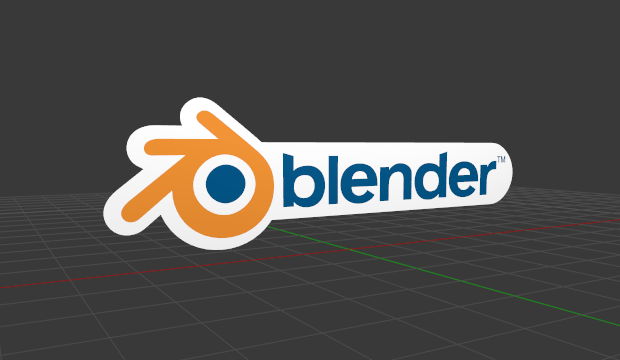# Introduction¶

Curves and Surfaces are particular types of Blender objects. They are expressed by mathematical functions (interpolation) rather than linear interpolation between a series of points.

Blender offers both Bézier and NURBS. Both Bézier curves and NURBS curves and surfaces are defined in terms of a set of “control points” (or “control vertices”) which define a “control polygon”.Blender logo made from Bézier curves.

Both Bézier and NURBS curves are named after their mathematical definitions, and choosing between them is often more a matter of how they are computed behind the scenes than how they appear from a modeler’s perspective. Bézier curves are generally more intuitive because they start and end at the control points that you set, but NURBS curves are more efficient for the computer to calculate when there are many twists and turns in a curve.

The main advantage to using curves instead of polygonal meshes is that curves are defined by less data and so can produce results using less memory and storage space at modeling time. However, this procedural approach to surfaces can increase demands at render time.

Certain modeling techniques, such as extruding a profile along a path, are possible only using curves. On the other hand, when using curves, vertex-level control is more difficult and if fine control is necessary, mesh editing may be a better modeling option.

Bézier curves are the most commonly used curves for designing letters or logos.

They are also widely used in animation, both as for objects to move along (see constraints below) and as F-Curves to change the properties of objects as a function of time.